NEET  >  Test: Fluids

# Test: Fluids - NEET

Test Description

## 30 Questions MCQ Test Physics Class 11 - Test: Fluids

Test: Fluids for NEET 2023 is part of Physics Class 11 preparation. The Test: Fluids questions and answers have been prepared according to the NEET exam syllabus.The Test: Fluids MCQs are made for NEET 2023 Exam. Find important definitions, questions, notes, meanings, examples, exercises, MCQs and online tests for Test: Fluids below.
Solutions of Test: Fluids questions in English are available as part of our Physics Class 11 for NEET & Test: Fluids solutions in Hindi for Physics Class 11 course. Download more important topics, notes, lectures and mock test series for NEET Exam by signing up for free. Attempt Test: Fluids | 30 questions in 60 minutes | Mock test for NEET preparation | Free important questions MCQ to study Physics Class 11 for NEET Exam | Download free PDF with solutions
 1 Crore+ students have signed up on EduRev. Have you?
Test: Fluids - Question 1

### As a bubble comes from the bottom of a lake to the top, its radius

Detailed Solution for Test: Fluids - Question 1

PV = constant

As a bubble comes from bottom to top , the pressure decrease from ( P0 + ρgh )  to P0

∴  Volume increases and hence radius increases.

Test: Fluids - Question 2

### To get the maximum flight, a ball must be thrown as

Detailed Solution for Test: Fluids - Question 2

Velocity of ball at top = V1 = VT + VCOM

Velocity of ball at bottom = V2 = | VT – VCOM |

V1 >> V2 than other option.

∴ More pressure difference is created because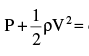constant

Test: Fluids - Question 3

### The tube shown is of uniform cross-section. Liquid flows through it at a constant speed in the direction shown by the arrows. The liquid exerts on the tube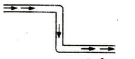Detailed Solution for Test: Fluids - Question 3

The forces exerted by the liquid at the bends are shown. (The liquid undergoes change of momentum only at these points, and hence the liquid and tube exert forces on each other.) The two forces form a couple exerting a clockwise torque.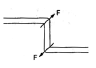Test: Fluids - Question 4

Water coming out of the mouth of a tap and falling vertically in streamline flow forms a tapering column, i.e., the area of cross-section of the liquid column decreases as it moves down. Which of the following is the most accurate explanation for this?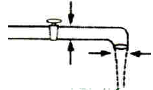Test: Fluids - Question 5

A cylindrical drum, open at the top, contains 30 litres of water. It drains out through a small opening at the bottom.10 litre of water comes out in time t, the next 10 litres in a further time t2 and the last 10 litres in a further time t3.Then

Detailed Solution for Test: Fluids - Question 5

We know that,
Velocity of efflux is given as v = √2gh
As the water level in the tank decreases, the velocity with which water comes out from the opening decreases due to decrease in pressure with height. Hence, the time taken to empty the tank increases with decrease in velocity and height of water level.
Hence C is the correct answer.

Test: Fluids - Question 6

The valve V in the bent tube is initially kept closed. Two soap bubbles A (smaller) and B (larger) are formed at the two open ends of the tube. V is now opened, and air can flow freely between the bubbles.

Test: Fluids - Question 7

Water rises in a straight capillary tube upto a height of 5 cm when held vertical in water. If the tube is bent as shown in figure then the height of water column in it will be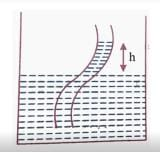Test: Fluids - Question 8

A piece of cork starts from rest at the bottom of a lake and floats up. Its velocity v is plotted against time t. Which of the following best represents the resulting curve?

Detailed Solution for Test: Fluids - Question 8

As the cork moves up, the force due to buoyancy remains constant. As its speed increases, the retarding force due to viscosity increases, being proportional to the speed. Thus. the acceleration gradually decreases. The acceleration is variable, and hence the relation between velocity and time is not linear.

Test: Fluids - Question 9

When cooking oil is heated in frying pan, the oil moves around in the pan more easily when it is hot. The main reason for this is that with rise in temperature, there is a decrease in

Test: Fluids - Question 10

A tank with vertical walls is mounted so that its base is at a height H above the horizontal ground. The tank is filled with water to the depth h. A hole is punched in the side wall of the tank at a depth x below the water surface. To have maximum range of the emerging stream, the value of x is

Detailed Solution for Test: Fluids - Question 10

To have the maximum range of the emerging stream, the value of x is to be (h/2) where h is the depth of water.

Test: Fluids - Question 11

The work done in increasing the volume of a soap bubble of radius r to 27 times will be, if the surface tension of soap solution is T

Detailed Solution for Test: Fluids - Question 11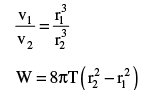Test: Fluids - Question 12

The excess pressure inside first soap bubble is 3 times that inside the second bubble. Then the ratio of volumes of the first and the second bubbles is

Detailed Solution for Test: Fluids - Question 12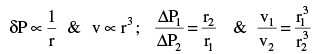Test: Fluids - Question 13

Water is rises to a height h1 in a capillary tube in a stationary lift. If the lift moves up with uniform acceleration it rises to a height h2, then acceleration of the lift is

Detailed Solution for Test: Fluids - Question 13

h2 (g + a) = h1 g

Test: Fluids - Question 14

A ball of mass ‘m’ and radius ‘r’ is released in viscous liquid. The value of its terminal velocity is proportional to

Detailed Solution for Test: Fluids - Question 14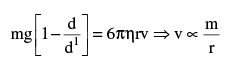Test: Fluids - Question 15

Viscosity is the property by virtue of which a liquid

Test: Fluids - Question 16

For an ideal fluid, viscosity is

Detailed Solution for Test: Fluids - Question 16

A fluid that has no resistance to shear stress is known as an ideal fluid.

Test: Fluids - Question 17

A liquid of density ρ and coefficient of viscosity η, flows with velocity v through a tube of diameter D. A quantity R = ρVD/ η determines whether the flow will be streamlined or turbulent. R has the dimension of

Test: Fluids - Question 18

Coefficient of viscosity of a gas

Test: Fluids - Question 19

Water flows through a frictionless duct with a cross-section varying as shown in figure. Pressure P at points along the axis is represented by (Assume water to be non-viscous)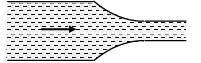Detailed Solution for Test: Fluids - Question 19

Application of Bernoulli’s theorem larger area of cross section implies less velocity which in turn implies higher pressure.

Test: Fluids - Question 20

There are two identical small holes on the opposite sides of a tank containing a liquid. The tank is open at the top. The difference in height between the two holes is h. As the liquid comes out of the two holes, the tank will experience a net horizontal force proportional to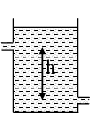Detailed Solution for Test: Fluids - Question 20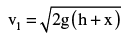Let ρ = density of the liquid.

Let α = area of a cross-section of each hole.

Volume of liquid discharged per second at a hole = αv.

Mass of liquid discharged per second = αvρ.

Momentum of liquid discharged per second = αv2ρ.

∴ the force exerted at the upper hole (to the right) = αρv22

and  the force exerted at the lower hole (to the left) = αρv12

Net force on the tank = 2αρgh.

Test: Fluids - Question 21

n drops of liquid, each with surface energy E, join to form a single drop. In this process

Detailed Solution for Test: Fluids - Question 21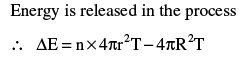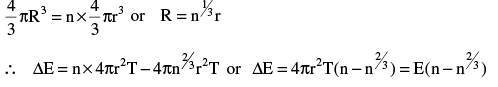Test: Fluids - Question 22

Two spherical soap bubbles of radii R1 and R2 combine under isothermal condition to form a single bubble. The radius of the resultant bubble is

Detailed Solution for Test: Fluids - Question 22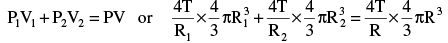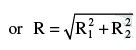Test: Fluids - Question 23

For the arrangement shown in figure, the time interval after which the water jet ceases to cross the wall (area of cross section of tank is A and orifice is ‘a’)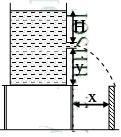Detailed Solution for Test: Fluids - Question 23

Velocity of efflux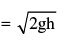Find ‘h’ to have range of ejected water ≥ x x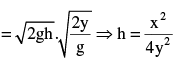Test: Fluids - Question 24

Two soap bubbles with radii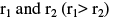come in contact. Their common surface has a radius of curvature r. Then

Detailed Solution for Test: Fluids - Question 24

Let p0 = atmospheric pressure,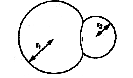p1 and p2 = pressures inside the two bubbles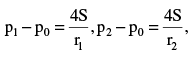Let r = radius of curvature of the common surface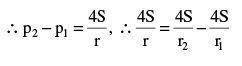Test: Fluids - Question 25

A constant height of 20 cm maintained in the contains water of 1 kg as shown in figure. A small orifice area 10-2 m2 is made at bottom to the vertical wall of container the ejected water is directed as shown in figure. Assuming mass of container is negligible, the net force on container is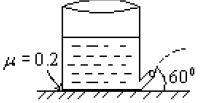Detailed Solution for Test: Fluids - Question 25

FBD of container: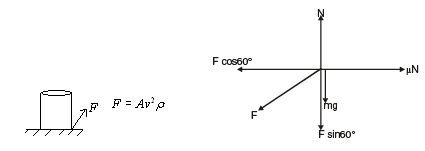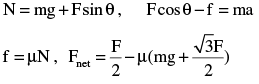Test: Fluids - Question 26

The surface of water in a water tank on the top of a house is 4 m above the tap level. The pressure of water at the tap when the tap is closed is (Density of water = 1000 kg/m3 g = 10 m/s2

Detailed Solution for Test: Fluids - Question 26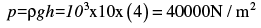Test: Fluids - Question 27

Water flows through a horizontal tube as shown in figure. If the difference of heights of water column in the vertical tubes is 60 m, and the areas areas of cross-section at A and B are 4m2 and 2 m2 respectively , find the rate of flow of water across any section is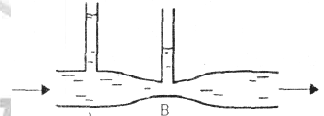Detailed Solution for Test: Fluids - Question 27

Using the following relations,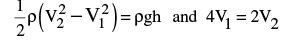We get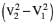= 2gh = 1200 and V2 = 2V1
Therefore, V1 = 20, V2 = 40m/s and the rate of flow of water = 80 m3/s

Test: Fluids - Question 28

A tube has two area of cross-sections as shown in figure. The diameters of the tube are 8 mm and 2 mm. Find range of water falling on horizontal surface, if piston is moving with a constant velocity of 0.25m/s, h = 1.25 m (g = 10 m/s2)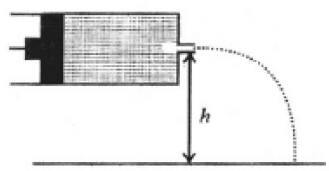Detailed Solution for Test: Fluids - Question 28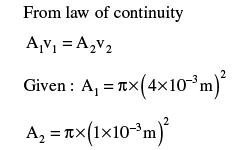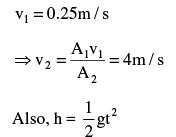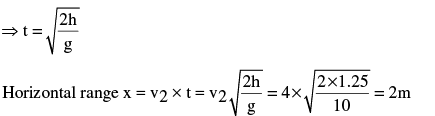Test: Fluids - Question 29

A large open tank has two holes in the wall. One is a square hole of side L at a depth y from the top and the other is a circular hole of radius R at a depth 4y from the top. When the tank is completely filled with water, the quantities of water lowing out per second  from both holes are the same. Then R is equal to

Detailed Solution for Test: Fluids - Question 29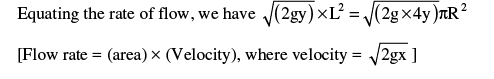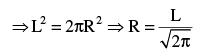Test: Fluids - Question 30

Water from a tap emerges vertically downwards with an initial speed of  1.0 m/s. The cross sectional area of the tap is 10-4 m2.Assume that the pressure is constant throughout the steam of water, and that the flow is steady. The cross sectional area of the stream 0.15 m below the tap is

Detailed Solution for Test: Fluids - Question 30

The equation of continuity is :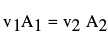where v and A represent the speed of water stream and its area of cross section, respectively. We are given that,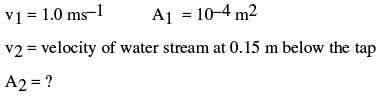For calculating v2

## Physics Class 11

130 videos|483 docs|210 tests(Scan QR code)